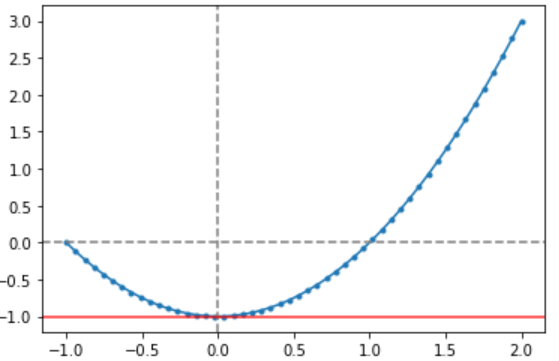## 高等数学之函数与极限

1、映射与函数

X:{0,1,2,3} Y:{0,2,4,6}; f x y y=f[x]=2x

``````#导入模块
import numpy as np
import pandas as dp
import matplotlib.pyplot as plt

#不发出警告
import warnings
warnings.filterwarnings('ignore')``````
``````#基本初等函数：三角函数
# f(x) = sin(ax)
x = np.linspace(-10,10,num = 50)
y = np.sin(x)

plt.scatter(x,y,marker='.')
plt.plot(x,y)

# 辅助线
plt.axvline(0,color = 'gray',linestyle = '--',alpha=0.8)
plt.axhline(0,color = 'gray',linestyle = '--',alpha=0.8)  ``````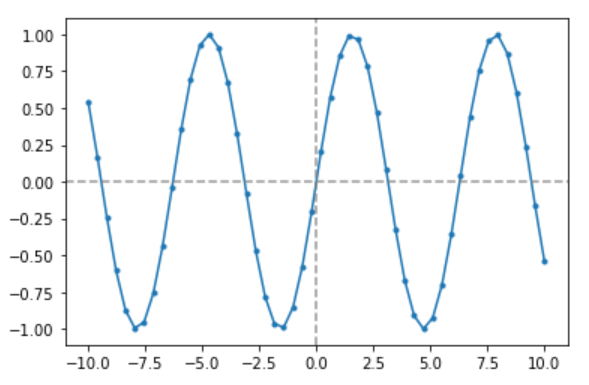``````#基本初等函数：反三角函数
# f(x) = arcsin(x)
x = np.linspace(-1,1,num = 50)
y = np.arcsin(x)

plt.scatter(x,y,marker='.')
plt.plot(x,y)

# 辅助线
plt.axvline(0,color = 'gray',linestyle = '--',alpha=0.8)
plt.axhline(0,color = 'gray',linestyle = '--',alpha=0.8) ``````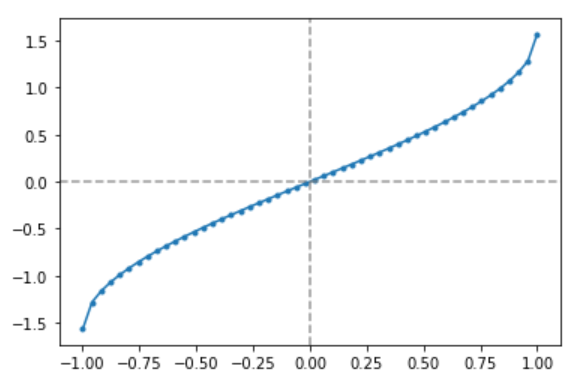``````# 基本初等函数：幂函数
# f(x) = x**a  比如a=2

x = np.linspace(-10,10,num = 50)
y = x**2

plt.scatter(x,y,marker='.')
plt.plot(x,y)

# 辅助线
plt.axvline(0,color = 'gray',linestyle = '--',alpha=0.8)
plt.axhline(0,color = 'gray',linestyle = '--',alpha=0.8) ``````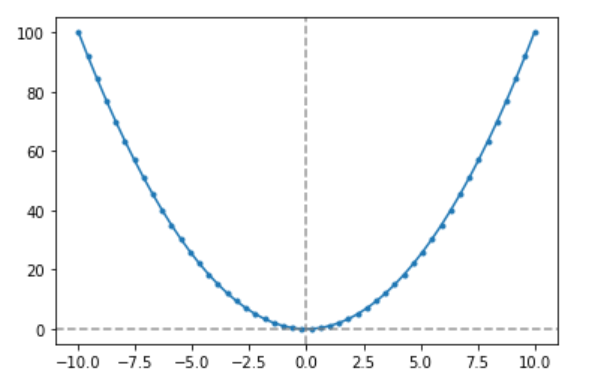``````# 基本初等函数：对数函数
# f(x) = loga(x)

x = np.linspace(-np.pi,2*np.pi,num = 50)
y = np.log2(x)

plt.scatter(x,y,marker = '.')
plt.plot(x,y)

plt.axvline(0,color = 'gray',linestyle = '--',alpha=0.8)
plt.axhline(0,color = 'gray',linestyle = '--',alpha=0.8) ``````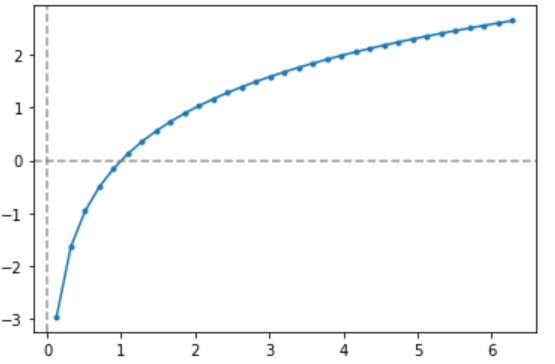2、数列及其极限

``````#生成一个含有11个数的数列
alist = []
for n in range(1,11):
alist.append('%i/%i' % (n,n+1))
alist  # 这里为了显示所以用字符串表示``````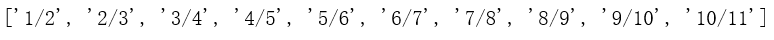``````x = np.arange(50)
y = x/(x+1)

plt.scatter(x,y,marker='.')
plt.plot(x,y)``````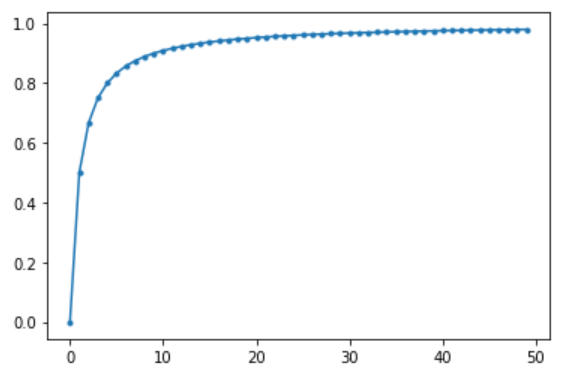3、函数的极限

``````x = np.linspace(-1,2,num=50)
y = x**2 - 1

plt.scatter(x,y,marker='.')
plt.plot(x,y)

plt.axvline(0,color = 'gray',linestyle = '--',alpha = 1)
plt.axhline(0,color = 'gray',linestyle = '--',alpha = 1)

plt.axhline(-1,color = 'red',alpha=0.8)
#x 趋于0时 函数极限值为-1``````